Download Presentation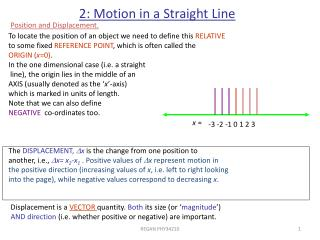2: Motion in a Straight Line

# 2: Motion in a Straight Line - PowerPoint PPT Presentation

The DISPLACEMENT, D x is the change from one position to another, i.e., D x= x 2 -x 1 . Positive values of D x represent motion in the positive direction (increasing values of x , i.e. left to right looking into the page), while negative values correspond to decreasing x. x =.I am the owner, or an agent authorized to act on behalf of the owner, of the copyrighted work described.
Download Presentation## 2: Motion in a Straight Line

An Image/Link below is provided (as is) to download presentation

Download Policy: Content on the Website is provided to you AS IS for your information and personal use and may not be sold / licensed / shared on other websites without getting consent from its author.While downloading, if for some reason you are not able to download a presentation, the publisher may have deleted the file from their server.

- - - - - - - - - - - - - - - - - - - - - - - - - - E N D - - - - - - - - - - - - - - - - - - - - - - - - - -
Presentation Transcript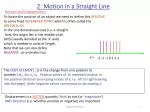The DISPLACEMENT, Dx is the change from one position to

another, i.e., Dx= x2-x1. Positive values of Dx represent motion in

the positive direction (increasing values of x, i.e. left to right looking

into the page), while negative values correspond to decreasing x.

x =

-3 -2 -1 0 1 2 3

2: Motion in a Straight Line

Position and Displacement.

To locate the position of an object we need to define this RELATIVE

to some fixed REFERENCE POINT, which is often called the

ORIGIN (x=0).

In the one dimensional case (i.e. a straight

line), the origin lies in the middle of an

AXIS (usually denoted as the ‘x’-axis)

which is marked in units of length.

Note that we can also define

NEGATIVE co-ordinates too.

Displacement is a VECTOR quantity. Both its size (or ‘magnitude’)

AND direction (i.e. whether positive or negative) are important.

REGAN PHY34210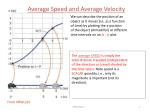Average Speed and Average Velocity

We can describe the position of an

object as it moves (i.e. as a function

of time) by plotting the x-position

of the object (Armadillo!) at different

time intervals on an (x , t) plot.

The average SPEED is simply the

total distance travelled (independent

of the direction or travel) divided by

the time taken. Note speed is a

SCALAR quantity, i.e., only its

magnitude is important (not its

direction).

From HRW p15

REGAN PHY34210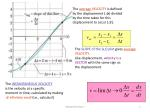The average VELOCITY is defined

by the displacement (Dx) divided

by the time taken for this

displacement to occur (Dt).

The SLOPE of the (x,t) plot gives average VELOCITY.

Like displacement, velocity is a

VECTOR with the same sign as

the displacement.

The INSTANTANEOUS VELOCITY

is the velocity at a specific

moment in time, calculated by making

Dt infinitely small (i.e., calculus!)

REGAN PHY34210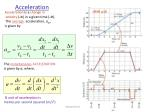The instantaneous ACCELERATION

is given by a, where,

SI unit of acceleration is

metres per second squared (m/s2)

Acceleration

HRW p18

Acceleration is a change in

velocity (Dv) in a given time (Dt).

The average acceleration, aav,

is given by

REGAN PHY34210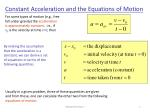By making the assumption

that the acceleration is a

constant, we can derive a set

of equations in terms of the

following quantities

Constant Acceleration and the Equations of Motion

For some types of motion (e.g., free

fall under gravity) the acceleration

is approximately constant, i.e., if

v0 is the velocity at time t=0, then

Usually in a given problem, three of these quantities are given

and from these, one can calculate the other two from the following

equations of motion.

REGAN PHY34210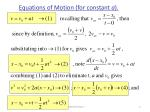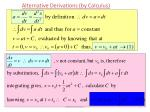Free-Fall Acceleration

At the surface of the earth, neglecting any effect due to air resistance

on the velocity, all objects accelerate towards the centre of earth

with the same constant value of acceleration.

This is called FREE-FALL ACCELERATION, or ACCELERATION

DUE TO GRAVITY, g.

At the surface of the earth, the magnitude of g = 9.8 ms-2

Note that for free-fall, the equations of motion are in the y-direction

(i.e., up and down), rather than in the x direction (left to right).

Note that the acceleration due to gravity is always towards the centre

of the earth, i.e. in the negative direction, a= -g = -9.8 ms-2

REGAN PHY34210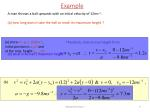(a) since a= -g = -9.8ms-2,

initial position is y0=0 and

at the max. height vm a x=0

Therefore, time to max height from

(b)

Example

A man throws a ball upwards with an initial velocity of 12ms-1.

(a) how long does it take the ball to reach its maximum height ?

(b) what’s the ball’s maximum height ?

REGAN PHY34210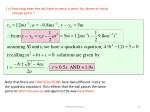release point ?

Note that there are TWO SOLUTIONS here (two different ‘roots’ to

the quadratic equation). This reflects that the ball passes the same

point on both the way up and again on the way back down.

REGAN PHY34210